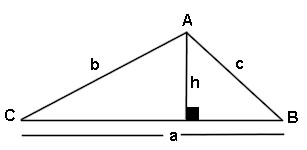# Triangle Area Side Side Side Calculator

This calculator requires the use of Javascript enabled and capable browsers. This calculator is designed to give the area of a triangle, assuming three sides and no angles are known, also known as SSS. Enter the three known sides. Then click on calculate. In a triangle, all interior angles total to 180 degrees. No two angles can total to 180 degrees or more. It is for any shape triangle. Click on Calculate. The three unknown angles and the area are returned. In case you need them, here are the Trig Triangle Formula Tables, as well as the Right Triangle Angle And Side Calculator. Here is our Triangle Math Menu.

 Unit Number Required Data Entry Quantity Decimal Places Angle Units Designation degrees (decimal) radians Known Side a Length Units Known Side b Length Units Known Side c Length Units Calculated Results Calculated Angle A Length Units Calculated Angle B Angle Units Calculated Angle C Angle Units Area Of Triangle Square UnitsUpdated 8.15.11

 Leave us a question or comment on FacebookSearch or Browse Our Site